# 14.11: Preparation of Organometallic Compounds

$$\newcommand{\vecs}{\overset { \rightharpoonup} {\mathbf{#1}} }$$ $$\newcommand{\vecd}{\overset{-\!-\!\rightharpoonup}{\vphantom{a}\smash {#1}}}$$$$\newcommand{\id}{\mathrm{id}}$$ $$\newcommand{\Span}{\mathrm{span}}$$ $$\newcommand{\kernel}{\mathrm{null}\,}$$ $$\newcommand{\range}{\mathrm{range}\,}$$ $$\newcommand{\RealPart}{\mathrm{Re}}$$ $$\newcommand{\ImaginaryPart}{\mathrm{Im}}$$ $$\newcommand{\Argument}{\mathrm{Arg}}$$ $$\newcommand{\norm}{\| #1 \|}$$ $$\newcommand{\inner}{\langle #1, #2 \rangle}$$ $$\newcommand{\Span}{\mathrm{span}}$$ $$\newcommand{\id}{\mathrm{id}}$$ $$\newcommand{\Span}{\mathrm{span}}$$ $$\newcommand{\kernel}{\mathrm{null}\,}$$ $$\newcommand{\range}{\mathrm{range}\,}$$ $$\newcommand{\RealPart}{\mathrm{Re}}$$ $$\newcommand{\ImaginaryPart}{\mathrm{Im}}$$ $$\newcommand{\Argument}{\mathrm{Arg}}$$ $$\newcommand{\norm}{\| #1 \|}$$ $$\newcommand{\inner}{\langle #1, #2 \rangle}$$ $$\newcommand{\Span}{\mathrm{span}}$$

## Metals with Organic Halides

The reaction of a metal with an organic halide is a convenient method for preparation of organometallic compounds of reasonably active metals such as lithium, magnesium, and zinc. Ethers, particularly diethyl ether and oxacyclopentane (tetrahydrofuran), provide inert, slightly polar media in which organometallic compounds usually are soluble. Care is necessary to exclude moisture, oxygen, and carbon dioxide, which would react with the organometallic compound. This can be accomplished by using an inert atmosphere of nitrogen or helium.The reactivity order of the halides is $$\ce{I} \: > \: \ce{Br} \: > \: \ce{Cl} \: \gg \ce{F}$$. Whereas magnesium and lithium react well with chlorides, bromides, and iodides, zinc is satisfactory only with bromides and iodides. Mercury only reacts when amalgamated with sodium. Sodium and potassium present special problems because of the high reactivity of alkylsodium and alkylpotassium compounds toward ether and organic halides. Alkane solvents usually are necessary.

Alkenyl, alkynyl, and aryl halides, like alkyl halides, can be converted to the corresponding magnesium and lithium compounds. However, the reaction conditions, such as choice of solvent, can be critical. Bromoethene, for instance, can be converted to ethenylmagnesium bromide in good yield if the solvent is oxacyclopentane [tetrahydrofuran, $$\ce{(CH_2)_4O}$$]: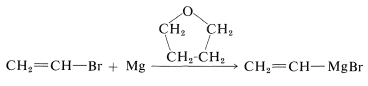The more reactive allylic and benzylic halides present a problem - not so much in forming the organometallic derivative as in keeping it from reacting further with the starting halide. An often unwanted side reaction in the preparation of organometallic compounds is a displacement reaction, probably of the $$S_\text{N}2$$ type: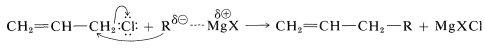This problem can be lessened greatly by using a large excess of magnesium and dilute solutions of the allylic halide to minimize the coupling reaction.

The same difficulty also occurs in the preparation of alkylsodium compounds. The starting halide $$\ce{RX}$$ couples with $$\ce{RNa}$$ (to give $$\ce{R-R}$$ and $$\ce{NaX}$$) or is converted to an alkene. These reactions appear to involve radical intermediates undergoing combination and disproportionation (Section 10-8C):In the absence of metallic sodium, ethylsodium probably still reacts with ethyl bromide by a radical reaction rather than $$S_\text{N}2$$ or $$E2$$. This happens because $$\ce{CH_3CH_2^-}$$ tends to lose an electron easily and can act like metallic sodium to donate an electron to $$\ce{CH_3CH_2Br}$$ to form an ethyl radical and itself become an ethyl radical: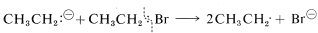Reactions between the resulting radicals then produce butane, ethane, and ethene.

The point at which one can expect $$S_\text{N}2$$ and $$E2$$ reactions to go faster than radical formation as the structures of the halides and the nature of the metal are changed is not yet clearly defined. However, it is becoming increasingly evident that there are substitution reactions of "unactivated" aryl halides that proceed without rearrangement by way of radical intermediates. The key step in these reactions is donation of an electron to one of the unfilled $$\pi$$ orbitals of the ring and subsequent ejection of a halide ion: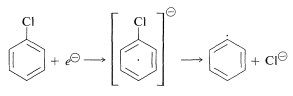Such a mechanism probably is involved in the formation of organometallic compounds from aryl halides and metals.

## Some Other Preparations of Organometallic Compounds

Brief descriptions follow of less general but very useful methods of forming organometallic compounds (also see Table 14-7). In each of these preparations the solvent must be inert to all of the organometallic compounds involved.

### Halogen-Metal Exchange

$\ce{RBr} + \ce{R'Li} \rightleftharpoons \ce{RLi} + \ce{R'Br}$

The equilibrium in these reactions favors formation of the organometallic compound with the metal attached to the more electronegative $$\ce{R}$$ group. The method is mainly used in the preparation of organolithium compounds derived from unreactive halides such as aryl, ethenyl, or ethynyl halides. These halides do not always react readily with lithium metal, but may react well with butyllithium: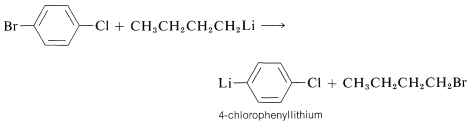### Displacement of One Metal by Another

$\ce{R_2Hg} + 2 \ce{Na} \rightleftharpoons 2 \ce{RNa} + \ce{Hg}$

Here the equilibrium is such that the $$\ce{R}$$ group favors attachment to the more electropositive metal.

### Organometallic Compounds with Metal Halides

$\ce{RMgCl} + \ce{HgCl_2} \rightleftharpoons \ce{RHgCl} + \ce{MgCl_2}$

$\ce{RLi} + \ce{CuI} \rightleftharpoons \ce{RCu} + \ce{LiI}$

The equilibrium favors the products with $$\ce{R}$$ connected to the less electropositive metal so the reaction tends to form a less reactive organometallic compound from a more reactive one.

### Organometallic Compounds from Acidic Hydrocarbons

Some organometallic compounds are prepared best by the reaction of a strong base or an alkyl metal derivative with an acidic hydrocarbon, such as an alkyne:An especially important example is that of 1,3-cyclopentadiene, which is acidic because its conjugate base (cyclopentadienide anion) is greatly stabilized by electron delocalization. The anion is formed easily from the hydrocarbon and methyllithium: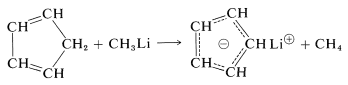## Organometallic Compounds from Polyhalogen Compounds

Diorganometallic compounds cannot be prepared from dihalides if the halogens are separated by three $$\ce{C-C}$$ bonds or less because elimination or other reactions usually predominate. With active metals and 1,1-, 1,2-, or 1,3-dihalides, the following reactions normally occur: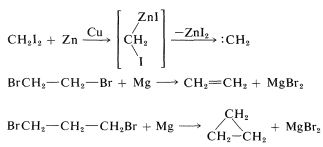When the halogens are at least four carbons apart a diorganometallic compound can be formed:Carbenes, $$\ce{R_2C} :$$ (Section 14-7B) are produced by $$\alpha$$ eliminations from polyhalogen compounds with organometallic reagents. The first step is halogen-metal exchange and this is followed by elimination of metal halide: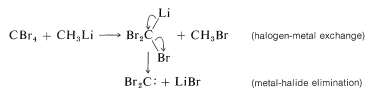Elimination reactions of this type can be useful in synthesis for the formation of carbon-carbon bonds. For example, if dibromocarbene is generated in the presence of an alkene, it will react by cycloaddition to give a cyclopropane derivative:A related example is the generation of benzyne from 1-bromo-2-fluorobenzene with magnesium in oxacyclopentane (tetrahydrofuran). If the temperature is kept around $$0^\text{o}$$, 2-fluorophenylmagnesium bromide is formed. At higher temperatures, magnesium halide is eliminated and benzyne results:If a diene is present, the benzyne will react with it by a [4 + 2] cycloaddition as in the following example: